# 4.1 Linear functions  (Page 16/27)

 Page 16 / 27

Write an equation for a line perpendicular to $\text{\hspace{0.17em}}h\left(t\right)=-2t+4\text{\hspace{0.17em}}$ and passing through the point $\text{\hspace{0.17em}}\left(-4,–1\right).$

Write an equation for a line perpendicular to $\text{\hspace{0.17em}}p\left(t\right)=3t+4\text{\hspace{0.17em}}$ and passing through the point $\text{\hspace{0.17em}}\left(3,1\right).$

$y=-\frac{1}{3}t+2$

## Graphical

For the following exercises, find the slope of the line graphed.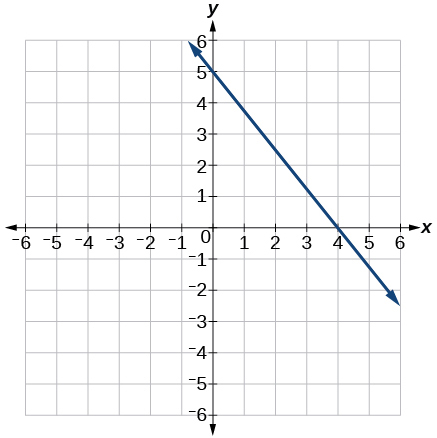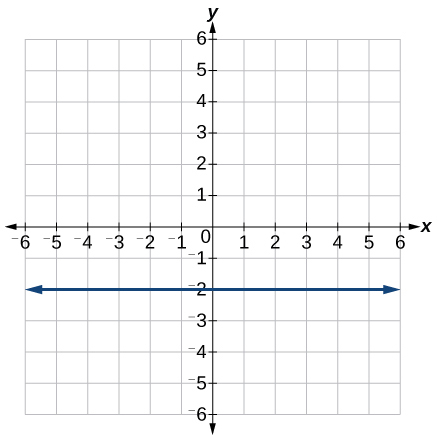0

For the following exercises, write an equation for the line graphed.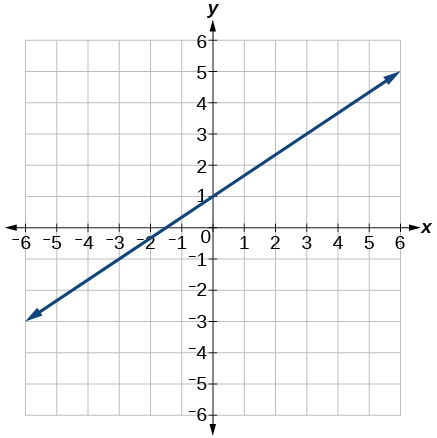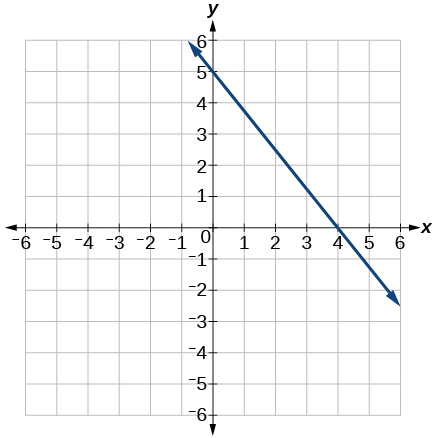$y=-\frac{5}{4}x+5$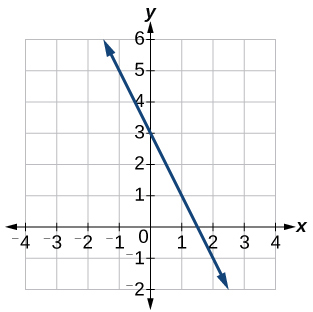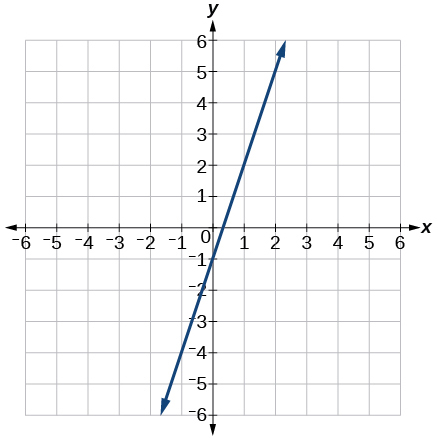$y=3x-1$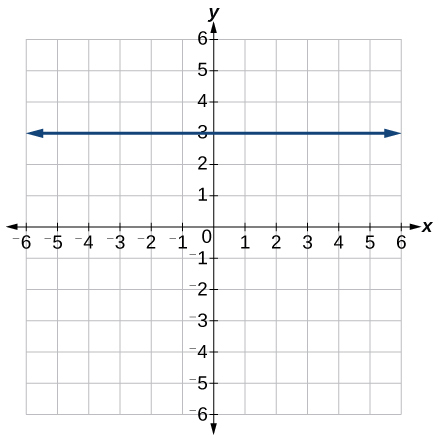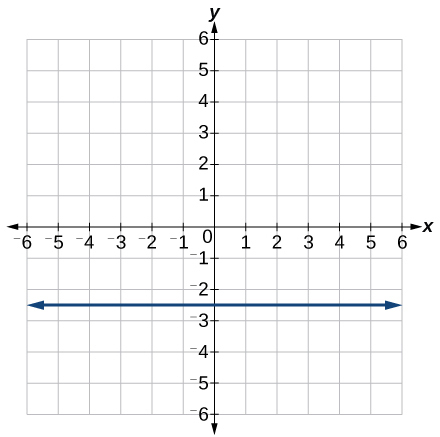$y=-2.5$

For the following exercises, match the given linear equation with its graph in [link] .

$f\left(x\right)=-x-1$

$f\left(x\right)=-2x-1$

F

$f\left(x\right)=-\frac{1}{2}x-1$

$f\left(x\right)=2$

C

$f\left(x\right)=2+x$

$f\left(x\right)=3x+2$

A

For the following exercises, sketch a line with the given features.

An x -intercept of $\text{\hspace{0.17em}}\left(–4,\text{0}\right)\text{\hspace{0.17em}}$ and y -intercept of $\text{\hspace{0.17em}}\left(0,\text{–2}\right)$

An x -intercept $\text{\hspace{0.17em}}\left(–2,\text{0}\right)\text{\hspace{0.17em}}$ and y -intercept of $\text{\hspace{0.17em}}\left(0,\text{4}\right)$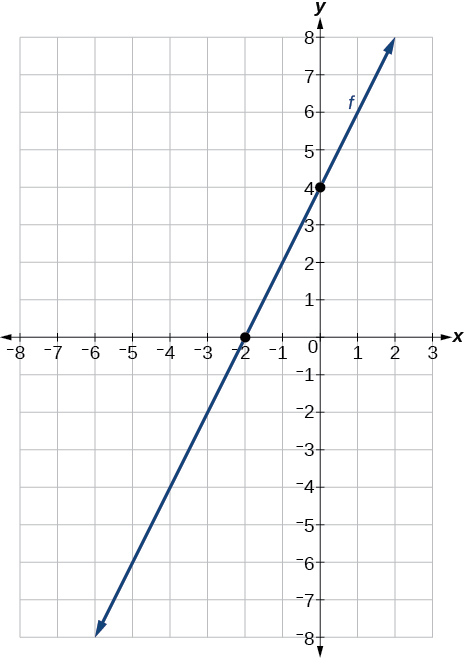A y -intercept of $\text{\hspace{0.17em}}\left(0,\text{7}\right)\text{\hspace{0.17em}}$ and slope $\text{\hspace{0.17em}}-\frac{3}{2}$

A y -intercept of $\text{\hspace{0.17em}}\left(0,\text{3}\right)\text{\hspace{0.17em}}$ and slope $\text{\hspace{0.17em}}\frac{2}{5}$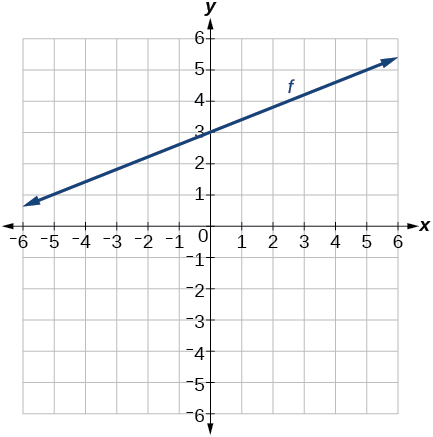Passing through the points $\text{\hspace{0.17em}}\left(–6,\text{–2}\right)\text{\hspace{0.17em}}$ and $\text{\hspace{0.17em}}\left(6,\text{–6}\right)$

Passing through the points $\text{\hspace{0.17em}}\left(–3,\text{–4}\right)\text{\hspace{0.17em}}$ and $\text{\hspace{0.17em}}\left(3,\text{0}\right)$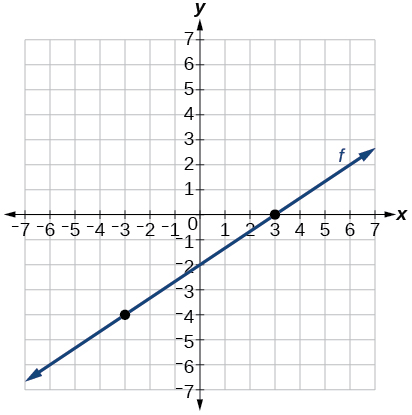For the following exercises, sketch the graph of each equation.

$f\left(x\right)=-2x-1$

$f\left(x\right)=-3x+2$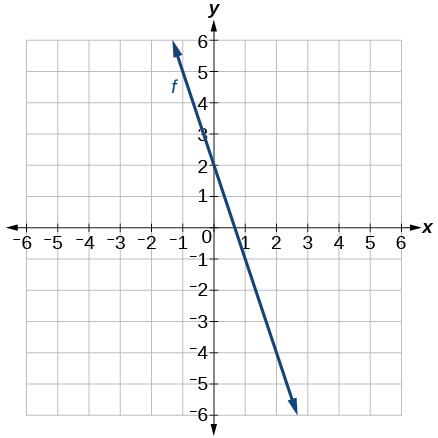$f\left(x\right)=\frac{1}{3}x+2$

$f\left(x\right)=\frac{2}{3}x-3$$f\left(t\right)=3+2t$

$p\left(t\right)=-2+3t$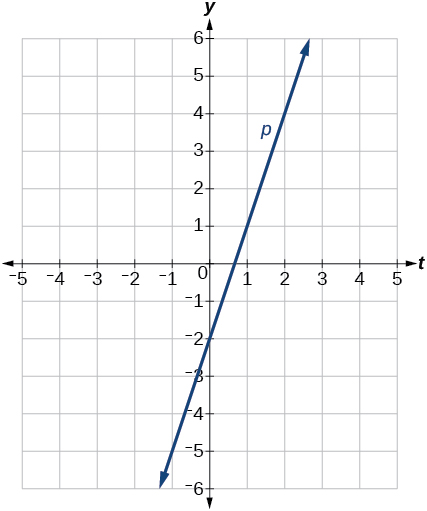$x=3$

$x=-2$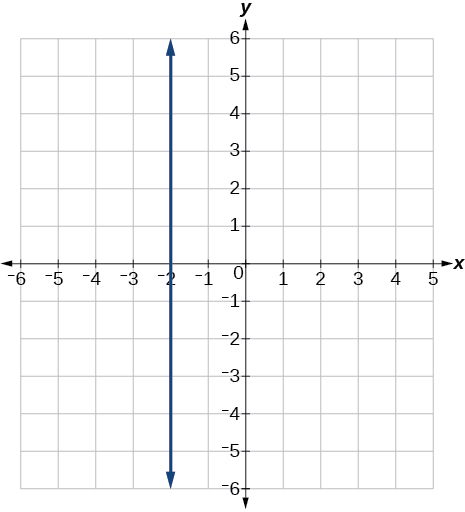$r\left(x\right)=4$

For the following exercises, write the equation of the line shown in the graph.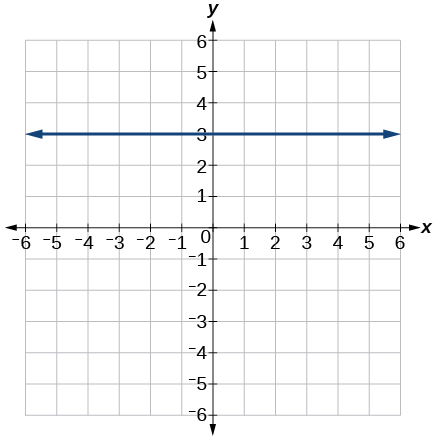$y=\text{3}$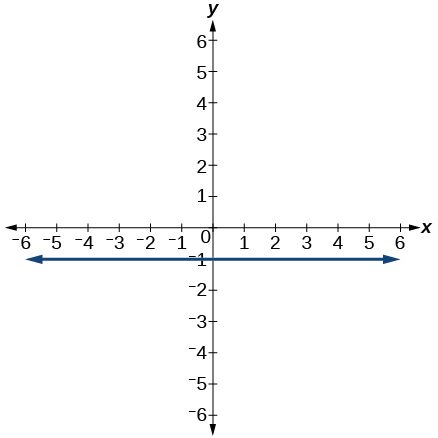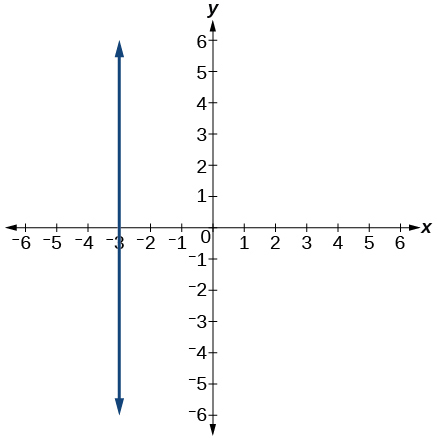$x=-3$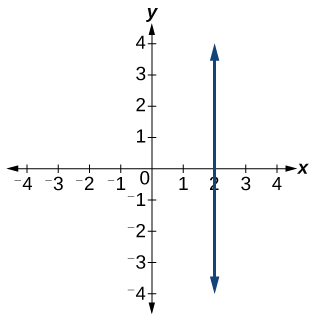## Numeric

For the following exercises, which of the tables could represent a linear function? For each that could be linear, find a linear equation that models the data.

 $x$ 0 5 10 15 $g\left(x\right)$ 5 –10 –25 –40

Linear, $\text{\hspace{0.17em}}g\left(x\right)=-3x+5$

 $x$ 0 5 10 15 $h\left(x\right)$ 5 30 105 230
 $x$ 0 5 10 15 $f\left(x\right)$ –5 20 45 70

Linear, $\text{\hspace{0.17em}}f\left(x\right)=5x-5$

 $x$ 5 10 20 25 $k\left(x\right)$ 13 28 58 73
 $x$ 0 2 4 6 $g\left(x\right)$ 6 –19 –44 –69

Linear, $\text{\hspace{0.17em}}g\left(x\right)=-\frac{25}{2}x+6$

 $x$ 2 4 8 10 $h\left(x\right)$ 13 23 43 53
 $x$ 2 4 6 8 $f\left(x\right)$ –4 16 36 56

Linear, $\text{\hspace{0.17em}}f\left(x\right)=10x-24$

 $x$ 0 2 6 8 $k\left(x\right)$ 6 31 106 231

## Technology

For the following exercises, use a calculator or graphing technology to complete the task.

If $\text{\hspace{0.17em}}f\text{\hspace{0.17em}}$ is a linear function, find an equation for the function.

$f\left(x\right)=-58x+17.3$

Graph the function $\text{\hspace{0.17em}}f\text{\hspace{0.17em}}$ on a domain of $\text{\hspace{0.17em}}\left[–10,10\right]:f\left(x\right)=0.02x-0.01.\text{\hspace{0.17em}}$ Enter the function in a graphing utility. For the viewing window, set the minimum value of $\text{\hspace{0.17em}}x\text{\hspace{0.17em}}$ to be $\text{\hspace{0.17em}}-10\text{\hspace{0.17em}}$ and the maximum value of $\text{\hspace{0.17em}}x\text{\hspace{0.17em}}$ to be $\text{\hspace{0.17em}}10.$

Graph the function $\text{\hspace{0.17em}}f\text{\hspace{0.17em}}$ on a domain of $\text{\hspace{0.17em}}\left[–10,10\right]:fx\right)=2,500x+4,000$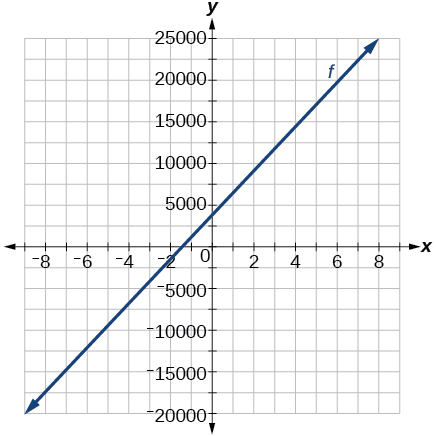[link] shows the input, $\text{\hspace{0.17em}}w,$ and output, $\text{\hspace{0.17em}}k,$ for a linear function $\text{\hspace{0.17em}}k.\text{\hspace{0.17em}}$ a. Fill in the missing values of the table. b. Write the linear function $\text{\hspace{0.17em}}k,$ round to 3 decimal places.

 w –10 5.5 67.5 b k 30 –26 a –44

[link] shows the input, $\text{\hspace{0.17em}}p,$ and output, $\text{\hspace{0.17em}}q,$ for a linear function $\text{\hspace{0.17em}}q.\text{\hspace{0.17em}}$ a. Fill in the missing values of the table. b. Write the linear function $\text{\hspace{0.17em}}k.$

 p 0.5 0.8 12 b q 400 700 a 1,000,000

Graph the linear function $\text{\hspace{0.17em}}f\text{\hspace{0.17em}}$ on a domain of $\text{\hspace{0.17em}}\left[-10,10\right]\text{\hspace{0.17em}}$ for the function whose slope is $\text{\hspace{0.17em}}\frac{1}{8}\text{\hspace{0.17em}}$ and y -intercept is $\text{\hspace{0.17em}}\frac{31}{16}.\text{\hspace{0.17em}}$ Label the points for the input values of $\text{\hspace{0.17em}}-10\text{\hspace{0.17em}}$ and $\text{\hspace{0.17em}}10.$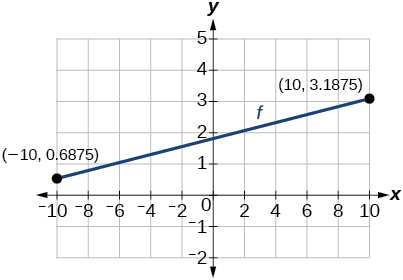Graph the linear function $\text{\hspace{0.17em}}f\text{\hspace{0.17em}}$ on a domain of $\text{\hspace{0.17em}}\left[-0.1,0.1\right]\text{\hspace{0.17em}}$ for the function whose slope is 75 and y -intercept is $\text{\hspace{0.17em}}-22.5.\text{\hspace{0.17em}}$ Label the points for the input values of $\text{\hspace{0.17em}}-0.1\text{\hspace{0.17em}}$ and $\text{\hspace{0.17em}}0.1.$

Graph the linear function $\text{\hspace{0.17em}}f\text{\hspace{0.17em}}$ where $\text{\hspace{0.17em}}f\left(x\right)=ax+b\text{\hspace{0.17em}}$ on the same set of axes on a domain of $\text{\hspace{0.17em}}\left[-4,4\right]\text{\hspace{0.17em}}$ for the following values of $\text{\hspace{0.17em}}a\text{\hspace{0.17em}}$ and $\text{\hspace{0.17em}}b.$

1. $a=2;b=3$
2. $a=2;b=4$
3. $a=2;b=–4$
4. $a=2;b=–5$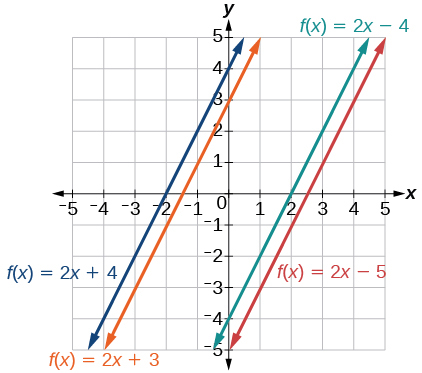## Extensions

Find the value of $\text{\hspace{0.17em}}x\text{\hspace{0.17em}}$ if a linear function goes through the following points and has the following slope: $\text{\hspace{0.17em}}\left(x,2\right),\left(-4,6\right),\text{\hspace{0.17em}}m=3$

Find the value of y if a linear function goes through the following points and has the following slope: $\text{\hspace{0.17em}}\left(10,y\right),\left(25,100\right),\text{\hspace{0.17em}}m=-5$

y = 175

By the definition, is such that 0!=1.why?
(1+cosA+IsinA)(1+cosB+isinB)/(cos@+isin@)(cos$+isin$)
hatdog
Mark
how we can draw three triangles of distinctly different shapes. All the angles will be cutt off each triangle and placed side by side with vertices touching
bsc F. y algebra and trigonometry pepper 2
given that x= 3/5 find sin 3x
4
DB
remove any signs and collect terms of -2(8a-3b-c)
-16a+6b+2c
Will
Joeval
(x2-2x+8)-4(x2-3x+5)
sorry
Miranda
x²-2x+9-4x²+12x-20 -3x²+10x+11
Miranda
x²-2x+9-4x²+12x-20 -3x²+10x+11
Miranda
(X2-2X+8)-4(X2-3X+5)=0 ?
master
The anwser is imaginary number if you want to know The anwser of the expression you must arrange The expression and use quadratic formula To find the answer
master
The anwser is imaginary number if you want to know The anwser of the expression you must arrange The expression and use quadratic formula To find the answer
master
Y
master
master
Soo sorry (5±Root11* i)/3
master
Mukhtar
2x²-6x+1=0
Ife
explain and give four example of hyperbolic function
What is the correct rational algebraic expression of the given "a fraction whose denominator is 10 more than the numerator y?
y/y+10
Mr
Find nth derivative of eax sin (bx + c).
Find area common to the parabola y2 = 4ax and x2 = 4ay.
Anurag
y2=4ax= y=4ax/2. y=2ax
akash
A rectangular garden is 25ft wide. if its area is 1125ft, what is the length of the garden
to find the length I divide the area by the wide wich means 1125ft/25ft=45
Miranda
thanks
Jhovie
What do you call a relation where each element in the domain is related to only one value in the range by some rules?
A banana.
Yaona
a function
Daniel
a function
emmanuel
given 4cot thither +3=0and 0°<thither <180° use a sketch to determine the value of the following a)cos thither
what are you up to?
nothing up todat yet
Miranda
hi
jai
hello
jai
Miranda Drice
jai
aap konsi country se ho
jai
which language is that
Miranda
I am living in india
jai
good
Miranda
what is the formula for calculating algebraic
I think the formula for calculating algebraic is the statement of the equality of two expression stimulate by a set of addition, multiplication, soustraction, division, raising to a power and extraction of Root. U believe by having those in the equation you will be in measure to calculate it
Miranda

#### Get Jobilize Job Search Mobile App in your pocket Now!ByByBy Stephen VoronBy Tod McGrathBy Alec MoffitBy OpenStaxBy Jazzycazz JacksonBy Jazzycazz JacksonBy Anh DaoByBy OpenStaxBy OpenStax